# Olympiad Test: Visualising Solid Shapes - 1

## 20 Questions MCQ Test Mathematical Olympiad Class 8 | Olympiad Test: Visualising Solid Shapes - 1

Description
Attempt Olympiad Test: Visualising Solid Shapes - 1 | 20 questions in 40 minutes | Mock test for Class 8 preparation | Free important questions MCQ to study Mathematical Olympiad Class 8 for Class 8 Exam | Download free PDF with solutions
QUESTION: 1

### A cube has ------ vertices.

Solution:

A cube has 8 vertices.

QUESTION: 2

### A cuboid has ------- edges.

Solution:

A cuboid has 12 edges.

QUESTION: 3

### A cube can be obtained by :

Solution:

A cube can be obtained by extruding a square.

QUESTION: 4

Which of the following is not a 3-D shape?

Solution:

A circle is not a 3-D shape.

QUESTION: 5

A sphere can be obtained by :

Solution:

A sphere can be obtained by rotating semicircle about its diameter.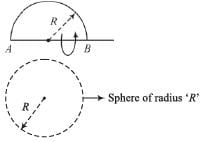QUESTION: 6

A polyhedron is such that its base and top are congruent polygons and other faces (lateral) are parallelograms in shape :

Solution:

Prism has congruent base and top faces and remaining faces are parallelograms.

QUESTION: 7

A regular polyhedron has :

Solution:

A regular polyhedron has congruent face.

QUESTION: 8

A cylinder has :

Solution:

A cylinder has 2 circular and 2 lateral surface.

QUESTION: 9

A tetrahedron has :

Solution:

A tetrahedron has 4 equilateral triangles.

QUESTION: 10

A hexahedron has :

Solution:

A hexahedron contains 6 squares.

QUESTION: 11

A paraboloid is formed by :

Solution:

A paraboloid can be generated by rotating a parabola about its axis.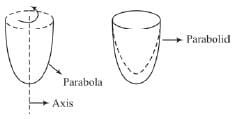QUESTION: 12

The number of edges of a octahedron are :

Solution:

Number of faces = F = 8
Number of vertices = V = 10
∴ Using Euler’s formula,
E = V  + F – 2 = 8 + 10 – 2 = 16

QUESTION: 13

A dodecahedron has 12 regular

Solution:

A dodecahedron has 12 regular pentagons.

QUESTION: 14

The number of faces of an icosahedron are :

Solution:

A isosahedron has 20 faces.

QUESTION: 15

A solid is formed by rotating right-angled triangle about any of its altitudes. The solid will be :

Solution: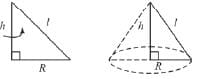QUESTION: 16

If two equal tetrahedrons are joined through their base triangles, by sticking, then the shape generated will be a :

Solution:

A hexahedron can be generated by sticking two equal tetrahedrons through their base.

*Multiple options can be correct
QUESTION: 17

While drawing an isometric view of a cube, the sides should be inclined at :

Solution: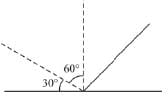The edges are inclined at 30° to the horizontal and 60° to the vertical

QUESTION: 18

The front view of a tetrahedron will be :

Solution: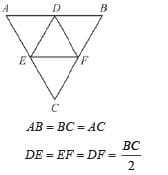[This is the front view of a tetrahedron]

QUESTION: 19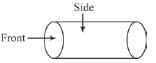The front and side views of a right circular cylinder are :

Solution: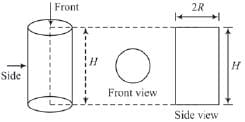QUESTION: 20

A solid shape is generated by rotating a rectangle about any of its sides. The shape will be :

Solution: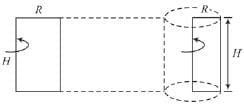Use Code STAYHOME200 and get INR 200 additional OFF Use Coupon Code